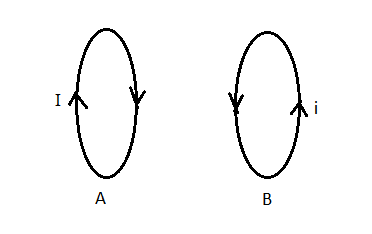# Two circular loops lie side by side in the same plane. One is connected to a source that supplies...

## Question:

Two circular loops lie side by side in the same plane. One is connected to a source that supplies an increasing current, the other is a simple closed ring.

a. Is the induced current in the ring in the same direction as that in the loop connected to the source connected or opposite?

b. What if the current in first loop is decreasing?

## Lenz's Law

As per Faraday's law whenever the magnetic flux linked with a coil changes, an electric current get induced in the coil, and the direction of the induced current should be such it opposes the cause producing it i.e. the induced current opposes the change in magnetic flux, this law is called Lenz's Law.

Let us draw a diagram of the situationPart A) Noif the current in the loop A is increasing the magnetic flux linked with the loop B also increases and according to the Lenz's law the current i induced in the loop B should be such that it tries to decrease the magnetic flux linked with it, means the magnetic field due to the induced current in loop B should be opposite to the magnetic field due to loop A, so the induced current in loop B must be in the opposite direction.

Part B) Yesif the current in the loop A is decreasing the magnetic flux linked with the loop B also decreases and according to the Lenz's law the current i induced in the loop B should be such that it tries to increase the magnetic flux linked with it, means the magnetic field due to the induced current in loop B should be in the same direction as the magnetic field due to loop A, so the induced current in loop B must be in the same direction.